# HSSlive: Plus One & Plus Two Notes & Solutions for Kerala State Board

## BSEB Class 9 Maths Chapter 1 Number Systems Ex 1.3 Textbook Solutions PDF: Download Bihar Board STD 9th Maths Chapter 1 Number Systems Ex 1.3 Book AnswersBSEB Class 9 Maths Chapter 1 Number Systems Ex 1.3 Textbook Solutions PDF: Download Bihar Board STD 9th Maths Chapter 1 Number Systems Ex 1.3 Book Answers

BSEB Class 9th Maths Chapter 1 Number Systems Ex 1.3 Textbooks Solutions and answers for students are now available in pdf format. Bihar Board Class 9th Maths Chapter 1 Number Systems Ex 1.3 Book answers and solutions are one of the most important study materials for any student. The Bihar Board Class 9th Maths Chapter 1 Number Systems Ex 1.3 books are published by the Bihar Board Publishers. These Bihar Board Class 9th Maths Chapter 1 Number Systems Ex 1.3 textbooks are prepared by a group of expert faculty members. Students can download these BSEB STD 9th Maths Chapter 1 Number Systems Ex 1.3 book solutions pdf online from this page.

## Bihar Board Class 9th Maths Chapter 1 Number Systems Ex 1.3 Books Solutions

 Board BSEB Materials Textbook Solutions/Guide Format DOC/PDF Class 9th Subject Maths Chapter 1 Number Systems Ex 1.3 Chapters All Provider Hsslive

## How to download Bihar Board Class 9th Maths Chapter 1 Number Systems Ex 1.3 Textbook Solutions Answers PDF Online?

2. Click on the Bihar Board Class 9th Maths Chapter 1 Number Systems Ex 1.3 Answers.
3. Look for your Bihar Board STD 9th Maths Chapter 1 Number Systems Ex 1.3 Textbooks PDF.
4. Now download or read the Bihar Board Class 9th Maths Chapter 1 Number Systems Ex 1.3 Textbook Solutions for PDF Free.

## BSEB Class 9th Maths Chapter 1 Number Systems Ex 1.3 Textbooks Solutions with Answer PDF Download

Find below the list of all BSEB Class 9th Maths Chapter 1 Number Systems Ex 1.3 Textbook Solutions for PDF’s for you to download and prepare for the upcoming exams:

## BSEB Bihar Board Class 9th Maths Solutions Chapter 1 Number Systems Ex 1.3

Question 1.
Write the following in decimal form and say what kind of decimal expansion each has :
(i) 36100
(ii) 111
(iii) 418
(iv) 211
(v) 211
(vi) 329400
Solution:
(i) 36100 = 0.36, terminating.

(ii) By long division, we have∴ 111 = 0.090909 = 0.09⎯⎯⎯⎯⎯⎯, non-terminating and repeating.

(iii) 418 = 4×8+18 = 338
By long division, we have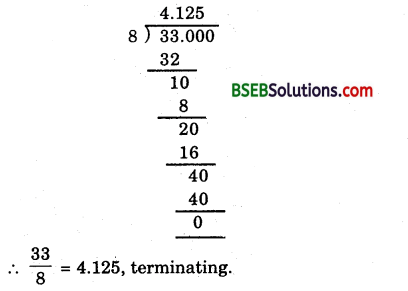(iv) By long division, we have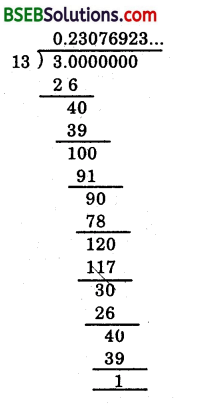∴311 = 0.23076923… = 0.230769⎯⎯⎯⎯⎯⎯⎯⎯⎯⎯⎯⎯⎯⎯⎯⎯⎯⎯⎯⎯⎯⎯, non-terminating and repeating.

(v) By long division, we have∴ 211 = 0.181818… = 0.18⎯⎯⎯⎯⎯⎯, non-terminating and repeating.

(vi) By long division, we have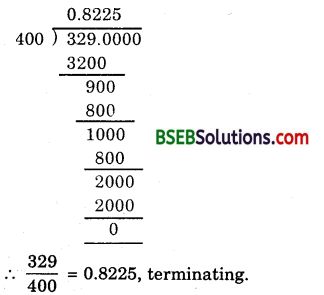Question 2.
You know that 17 = 0.142857⎯⎯⎯⎯⎯⎯⎯⎯⎯⎯⎯⎯⎯⎯⎯⎯⎯⎯. Can you predict what the decimal expansions of 27, 37, 47, 57, 67 are, without actually doing the long division? If so, how?
Solution:
Yes. All of the above will have repeating decimals which are permutations of 1, 4, 2, 8, 5, 7.
For example, here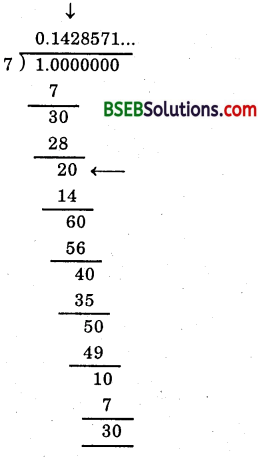To find 27, locate when the remainder becomes 2 and respective quotient (here it is 2), then write the, new quotient beginning from there (the arrows drawn in the above division) using the repeating digits 1, 4, 2, 8, 5, 7.Question 3.
Express the following in the form 𝑝𝑞, where p and q are integers and q ≠ 0.
(i) 0.6¯
(ii) 0.47¯
(iii) 0.001¯
Solution:
(i) Let x = 0.6¯
Then, x = 0.666 … (1)
Here, we have only one repeating digit. So, we multiply both sides of (1) by 10 to get
10x = 6.66 … (2)
Subtracting (1) from (2), we get
10x – x = (6.66…) – (0.66…)
⇒ 9x = 6 ⇒ x = 23
Hence, 0.6¯ = 23

(ii) Let x = 0.47¯
Clearly, there is just one digit on the right side of the decimal point which is without bar. So, we multiply both sides by x so that only the repeating decimal is left on the right side of the decimal point.
∴ 10x = 4.7¯
⇒ 10x = 4 + 0.7¯
⇒ 10x = 4 + 79

Rule :

• Write the number without decimal point as numerator.
• Write as many 9’s as there are different repeating digits for the denominator.

For example : 0.7¯ = 79, 0.35⎯⎯⎯⎯⎯⎯ = 3599 etc.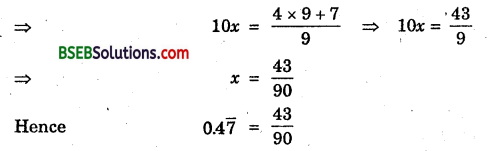Aliter:
Let x = 0.47¯ = 0.4777 …
∴ 10x = 4.777 …. (1)
and 100x = 47.777 …. (2)
Subtracting (1) from (2), we get
100x – 10x = (47.777…) – (4.777…)
⇒ 90x = 43 ⇒ x = 4390
Hence, 0.47¯ = 4390

(iii) Let x = 0.001⎯⎯⎯⎯⎯⎯⎯⎯⎯
⇒ x = 0.001001001 … (1)
Here, we have three repeating digits after the decimal point. So, we multiply (1) by 10³ = 1000, we get
1000x = 1.001001 … (2)
Subtracting (1) from (2), we get
1000x – x = (1001001 …) – (0.001001…)
⇒ 999x = 1 ⇒ x = 1999
Hence, 0.001⎯⎯⎯⎯⎯⎯⎯⎯⎯1999

Question 4.
Express 0.99999 …. in the form 𝑝𝑞. Are you surprised by your answer? With your teacher and classmates discuss why the answer makes sense.
Solution:
Let x = 0.9999 … (1)
Here, we have only one repeating digit. So, we multiply both sides of (1) by 10 to get
10x = 9.999 …(2)
Subtracting (1) from (2), we get
10x – x = (9.999…) – (0.999…)
⇒ 9x = 9 ⇒ x = 1
Hence, 0.9999… = 1
Since 0.9999… goes on forever. So, there is no gap between 1 and 0.9999… and hence they are equal.

Question 5.
What can the maximum number of digits be in the repeating block of digits in the decimal expansion 117? Perform the division to check your answer.
Solution:
We have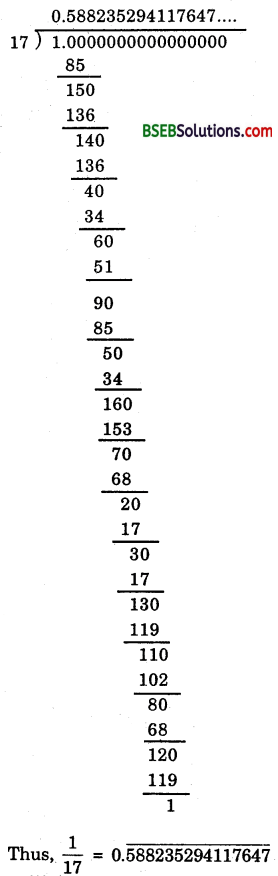∴ The maximum number of digits in the quotient while computing 117 are 15.

Question 6.
Look at several examples of rational numbers in the form 𝑝𝑞(q ≠ 0), where p and q are integers with no common factors other than 1 and having terminating decimal representations (expansions). Can you guess what property q must satisfy?
Solution:
Consider several rational numbers in the form 𝑝𝑞 (q ≠ 0), where p and q are integers with common factors other than 1 and having terminating decimal representation.
Let the various such rational numbers be 12, 14, 78, 3725, 8125, 1720, 3116 etc.
In all cases, we think of the natural number which when multipled by their respective denominators gives 10 or a power of 10.We have seen that those rational numbers whose denominators when multiplied by a suitable integer produce a power of 10 are expressible in the finite decimal form. But this can always be done only when the denominator of the given rational number has either 2 or 5 or both of them as the only prime factors. Thus, we obtain the following property :
If the denominator of a rational number in standard form has no prime factors other than 2 or 5, then and only then it can be represented as a terminating decimal.

Question 7.
Write three numbers whose decimal expansions are non-terminating non-recurring.
Solution:
Three numbers whose decimal representations are non-terminating and non-repeating are 2‾√, 3‾√ and 5‾√ or we can say 0.100100010001…, 0.20200200020002… and .003000000003…

Question 8.
Find three different irrational numbers between the rational numbers 57 and 911.
Solution:
We haveThus, three different irrational numbers between 57 and 911 are
0. 75075007500075000075…, 0.767076700767000… and 0.80800800080000…

Question 9.
Classify the following numbers as rational or irrational :
(i) 23‾‾‾√
(ii) 225‾‾‾‾√
(iii) 0.3796
(iv) 7.478498 …
(v) 1.101001000100001…
Solution:
(i) 23‾‾‾√ is an irrational number as 23 is not a perfect square.
(ii)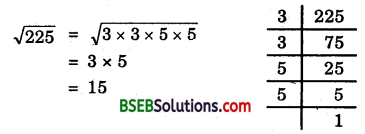Thus, 15 is a rational number.
(iii) 0.3796 is a rational number as it is terminating decimal.
(iv) 7.478478… is non – terminating but repeating, so, it is a rational number.
(v) 1.101001000100001… is non-terminating and non-repeating so, it is an irrational number.

## Bihar Board Class 9th Maths Chapter 1 Number Systems Ex 1.3 Textbooks for Exam Preparations

Bihar Board Class 9th Maths Chapter 1 Number Systems Ex 1.3 Textbook Solutions can be of great help in your Bihar Board Class 9th Maths Chapter 1 Number Systems Ex 1.3 exam preparation. The BSEB STD 9th Maths Chapter 1 Number Systems Ex 1.3 Textbooks study material, used with the English medium textbooks, can help you complete the entire Class 9th Maths Chapter 1 Number Systems Ex 1.3 Books State Board syllabus with maximum efficiency.

## FAQs Regarding Bihar Board Class 9th Maths Chapter 1 Number Systems Ex 1.3 Textbook Solutions

#### Can we get a Bihar Board Book PDF for all Classes?

Yes you can get Bihar Board Text Book PDF for all classes using the links provided in the above article.

## Important Terms

Bihar Board Class 9th Maths Chapter 1 Number Systems Ex 1.3, BSEB Class 9th Maths Chapter 1 Number Systems Ex 1.3 Textbooks, Bihar Board Class 9th Maths Chapter 1 Number Systems Ex 1.3, Bihar Board Class 9th Maths Chapter 1 Number Systems Ex 1.3 Textbook solutions, BSEB Class 9th Maths Chapter 1 Number Systems Ex 1.3 Textbooks Solutions, Bihar Board STD 9th Maths Chapter 1 Number Systems Ex 1.3, BSEB STD 9th Maths Chapter 1 Number Systems Ex 1.3 Textbooks, Bihar Board STD 9th Maths Chapter 1 Number Systems Ex 1.3, Bihar Board STD 9th Maths Chapter 1 Number Systems Ex 1.3 Textbook solutions, BSEB STD 9th Maths Chapter 1 Number Systems Ex 1.3 Textbooks Solutions,
Share: